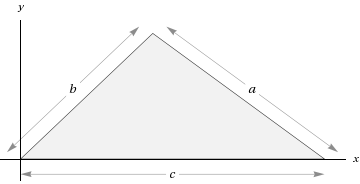I have forgotten

•http://facebook.com/
•https://www.google.com/accounts/o8/id
•https://me.yahoo.com

# Definitions

Describes the abbreviationsView other versions (2)

## Geometry

Geometry is one of the oldest branches of mathematics .
The word geometry comes from an ancient greek word which can be translated as "Earth-measuring". In conclusion geometry is a mathematical branch concerned with questions of size (areas, volumes etc), shape (triangle,square,cube etc) and positions of points(middle, the first point, center of gravity etc) or figures in an Euclidean space.

The field of astronomy, especially mapping the positions of the stars and planets on the celestial sphere and describing the relationship between movements of celestial bodies, served as an important source of geometric problems during the next one and a half millennia.

### Point

In geometry a point is a primitive notion upon which other concepts may be defined. In geometry, points have neither volume, area, length, nor any other higher dimensional analogue.
Thus, a point is a 0-dimensional object. A point could also be defined as a sphere which has a diameter of zero (or a circle with a 0 diameter).

### Line

The notion of line or straight line was introduced by the ancient mathematicians (Euclidean geometry) to represent straight objects with negligible width and depth. Euclid (ancient greek mathematician) described a line as "breadthless length".

Note
The line has the equationThe length of a straight line is infinity.

### Triangle

A triangle a shape formed with three corners or vertices and three sides or edges which are line segments.
A triangle with vertices,, andis denoted.
In Euclidean geometry any three non-collinear points determine a unique triangle and a unique plane (i.e. a two-dimensional Euclidean space).Triangles can be classified according to the relative lengths of their sides in :
• equilateral triangle, if.
• isosceles triangle if two sides are equal in length.

• scalene triangle if all sides are unequal.

Triangles can also be classified according to their internal angles in :
• right triangle, if has one of its interior angles measuring 90 degrees.

• acute triangle, if all of its angles are less then 90 degrees.

• obtuse triangle, if has one of its angles greater then 90 degrees.

### Circle

A circle is a simple shape of Euclidean geometry consisting of those points in a plane which are equidistant from a given point, called the centre .
A circle's diameter is the length of a line segment whose endpoints lie on the circle and which passes through the centre.
The radius is half the diameter of the circle.

### Square

A square is a regular quadrilateral.
This means that it has four equal sides and four equal angles (90 degree angles, or right angles).
A square with vertices ABCD would be denoted.

The area of a square iswhereis the side of the square.
The perimeter of a square iswhereis the side of the square.

## Analytic Geometry

In mathematics, analytic geometry, also known as coordinate geometry, or Cartesian geometry, is the study of geometry using a coordinate system and the principles of algebra and analysis.

This contrasts with the synthetic approach of Euclidean geometry, which treats certain geometric notions as primitive, and uses deductive reasoning based on axioms and theorems to derive truth.
Analytic geometry is widely used in physics and engineering, and is the foundation of most modern fields of geometry, including algebraic, differential, discrete, and computational geometry.

### Coordinate Geometry

A coordinate system or a Carthesian system is a system which uses one or more numbers, or coordinates, to uniquely determine the position of a point or other geometric element.
For example in a two dimensional space a point can be uniquely determined by a pair.

## Non-euclidean Geometries

A non-Euclidean geometry is the study of shapes and constructions that do not map directly to any n-dimensional Euclidean system

For example :
• hyperbolic geometry or Lobachevskian geometry
• elliptic geometry